# Marginal propensity to save

Jump to: navigation, search

## Definition

The marginal propensity to save (denoted MPS) for an individual or household is a dimensionless quantity that measures the fraction of an additional unit of disposable income (i.e., the residual income after taxes and transfers) that is allocated to savings. Note that the marginal propensity to save may not be a constant but may itself vary based on the level of disposable income.

The underlying additive identity at the heart of this concept is: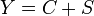$\! Y = C + S$

where$Y$ denotes disposable income,$C$ is the amount allocated to consumption, and$S$ is the amount allocated to saving.

### Differential definition

This definition measures the marginal propensity to save at a given level of disposable income, and is defined as the derivative of saving with respect to disposable income. If$Y$ denotes disposable income and$S$ denotes the total money allocated to saving, the marginal propensity to save is given by: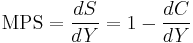$\operatorname{MPS} = \frac{dS}{dY} = 1 - \frac{dC}{dY}$

Note that the value may not be a constant but may depend on the level of$Y$.

### Interval definition

This definition measures the marginal propensity to consume between two levels of disposable income, and is defined as the difference quotient of saving with respect to disposable income. If the consumption level is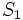$S_1$ for a disposable income of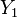$Y_1$ and is$S_2$ for a disposable income of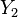$Y_2$, the marginal propensity to consume is given by:$\operatorname{MPS} = \frac{S_2 - S_1}{Y_2 - Y_1} = \frac{\Delta S}{\Delta Y} = 1 - \frac{\Delta C}{\Delta Y}$

The two definitions are related in that the derivative is a limit of a difference quotient, so as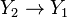$Y_2 \to Y_1$, we obtain the marginal propensity to save at$Y_1$.

## Values and interpretations

Value of MPS What it means
Greater than 1 At the margin, a slight increase in disposable income leads to a decrease in consumption and an increase in savings. This is fairly rare, but plausible, for instance, if an increase in income gives the person more hope for the future and thus shift to a longer time horizon, making saving more attractive.
1 At the margin, none of the additional disposable income is allocated to consumption, and all of it is saved. In other words, total consumption is (locally) constant with respect to income. This indicates that the person is sated with respect to consumption in the short run,
Between 0 and 1 At the margin, some of the additional disposable income is allocated to consumption, and some of it is saved. Both the quantity consumed and the quantity saved increase.
0 At the margin, all additional disposable income is allocated to consumption, and none of it is saved. In other words, total saving is (locally) constant with respect to income.
Greater than 0 At the margin, a given increase in income results in a greater increase in consumption. This may happen if an increase in income is an increase in permanent income, so it makes the person more confident and less worried about meeting future needs, and hence the person feels less of a need to save.

## Related notions

• Marginal propensity to consume, denoted MPC, is defined as$1 - MPS$, and is the fraction of additional disposable income allocated to additional savings.
• Average propensity to consume, denoted APC, is defined as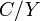$C/Y$, and is the fraction of total disposable income spent on consumption.
• Average propensity to save, denoted APS, is defined as$1 - APC$, and is the fraction of total disposable income allocated to savings.

## Short run versus long run

Some part of consumption decisions is made based on the (estimate of) long run disposable income, also called permanent income. Other consumption decisions are made based on short run disposable income. It is possible to conceptually distinguish the marginal propensity to save based on permanent income from the marginal propensity to save based on short run income.